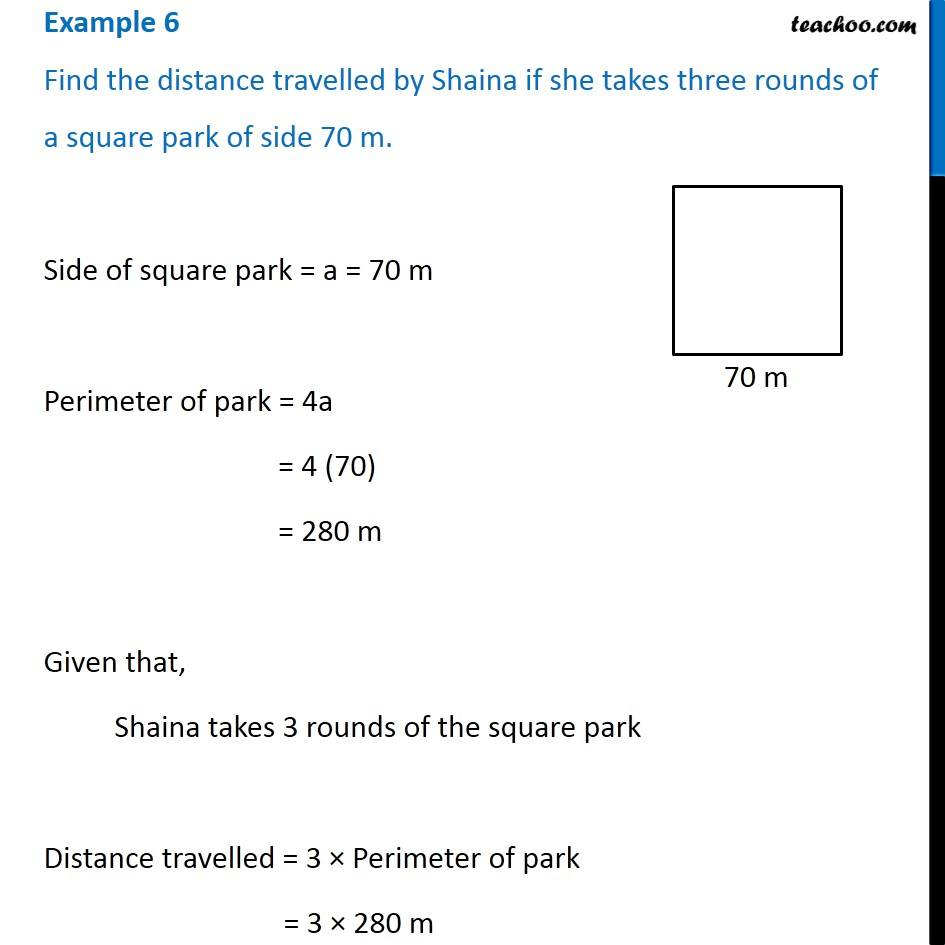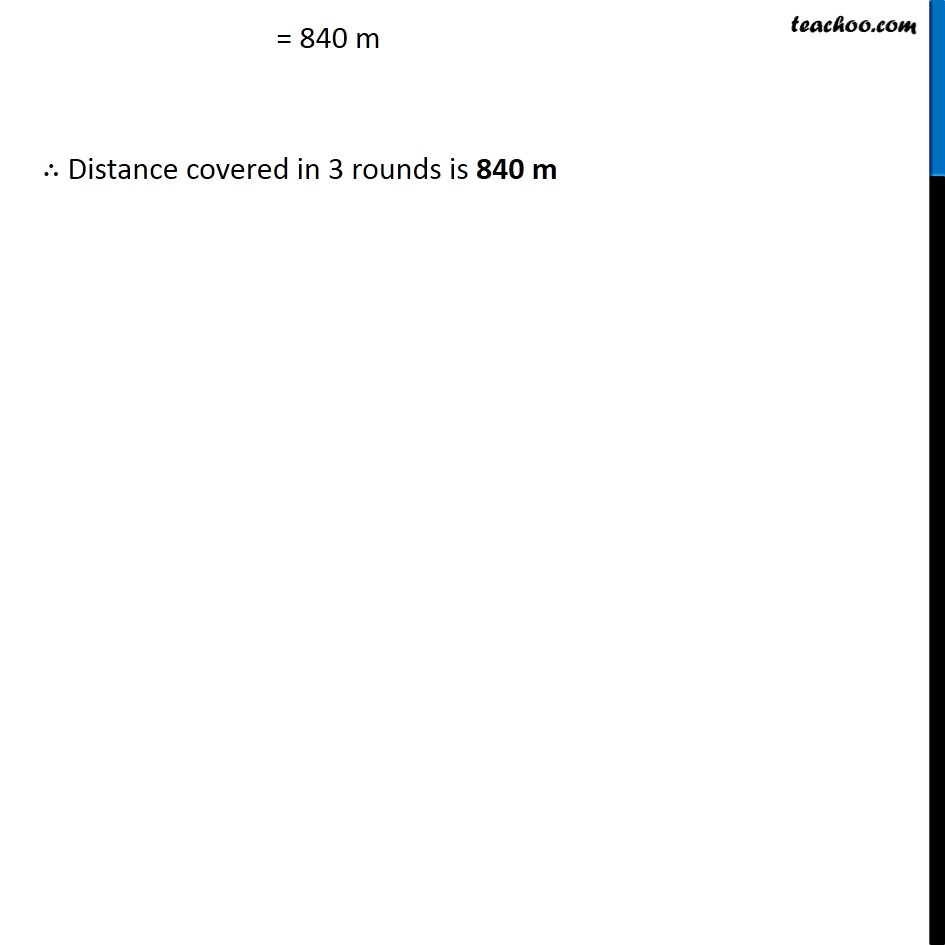Examples

Chapter 10 Class 6 Mensuration
Serial order wiseLearn in your speed, with individual attention - Teachoo Maths 1-on-1 Class

### Transcript

Example 6 Find the distance travelled by Shaina if she takes three rounds of a square park of side 70 m. Side of square park = a = 70 m Perimeter of park = 4a = 4 (70) = 280 m Given that, Shaina takes 3 rounds of the square park Distance travelled = 3 × Perimeter of park = 3 × 280 m = 840 m ∴ Distance covered in 3 rounds is 840 m